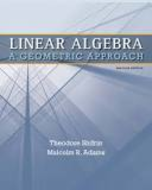Linear Algebra: A Geometric Approach - 2 Edition - Chapter 7.1 - Problem 6
Register Now

Join StudySoup

Get Full Access to Linear Algebra: A Geometric Approach - 2 Edition - Chapter 7.1 - Problem 69781429215213

# Prove that if p(t) = (t )3 and dim N(A I) = 1, then we must have N(A I) C(A I). (Hint

Linear Algebra: A Geometric Approach | 2nd Edition

Problem 6

Prove that if p(t) = (t )3 and dim N(A I) = 1, then we must have N(A I) C(A I). (Hint: If N(A I) C(A I) = {0}, use the twodimensional case already proved to deduce that dim N(A I) 2.)

Accepted Solution
Step-by-Step Solution:

Step 1 of 2

It is given that,.

And,.

To prove that,.

###### Chapter 7.1, Problem 6 is Solved

Step 2 of 2

Unlock Textbook Solution